Inverse of a function

Chapter 1 Class 12 Relation and Functions
Serial order wise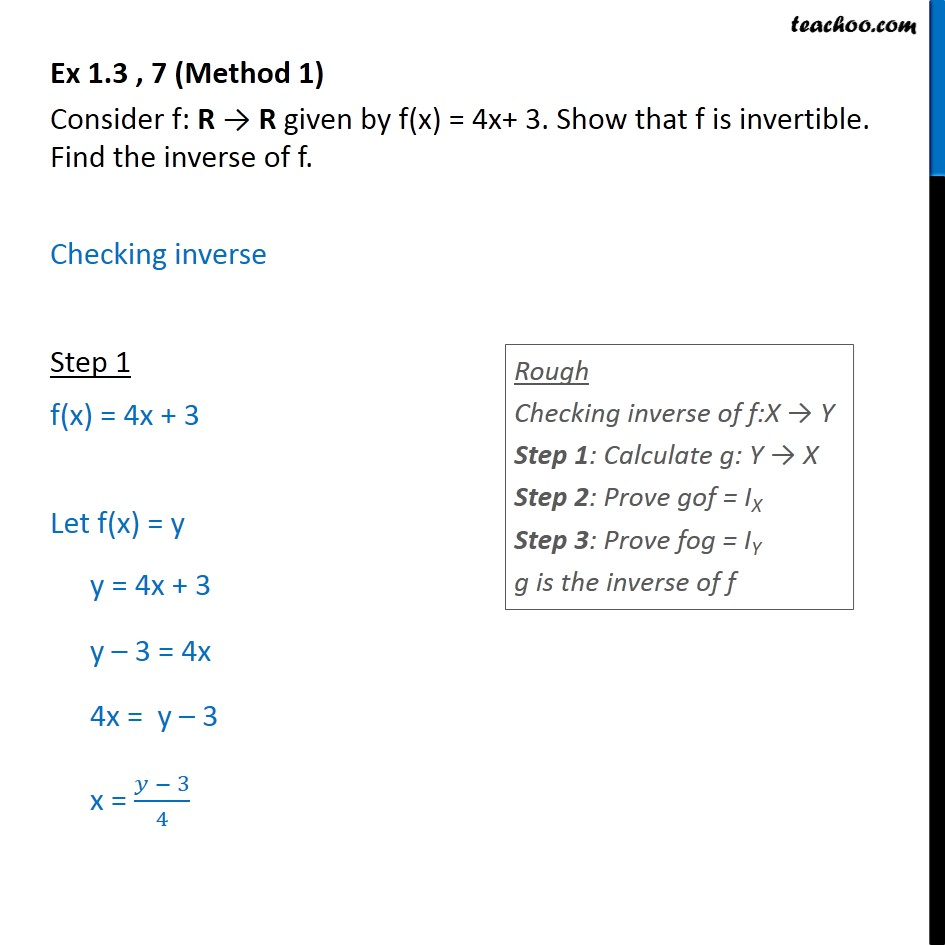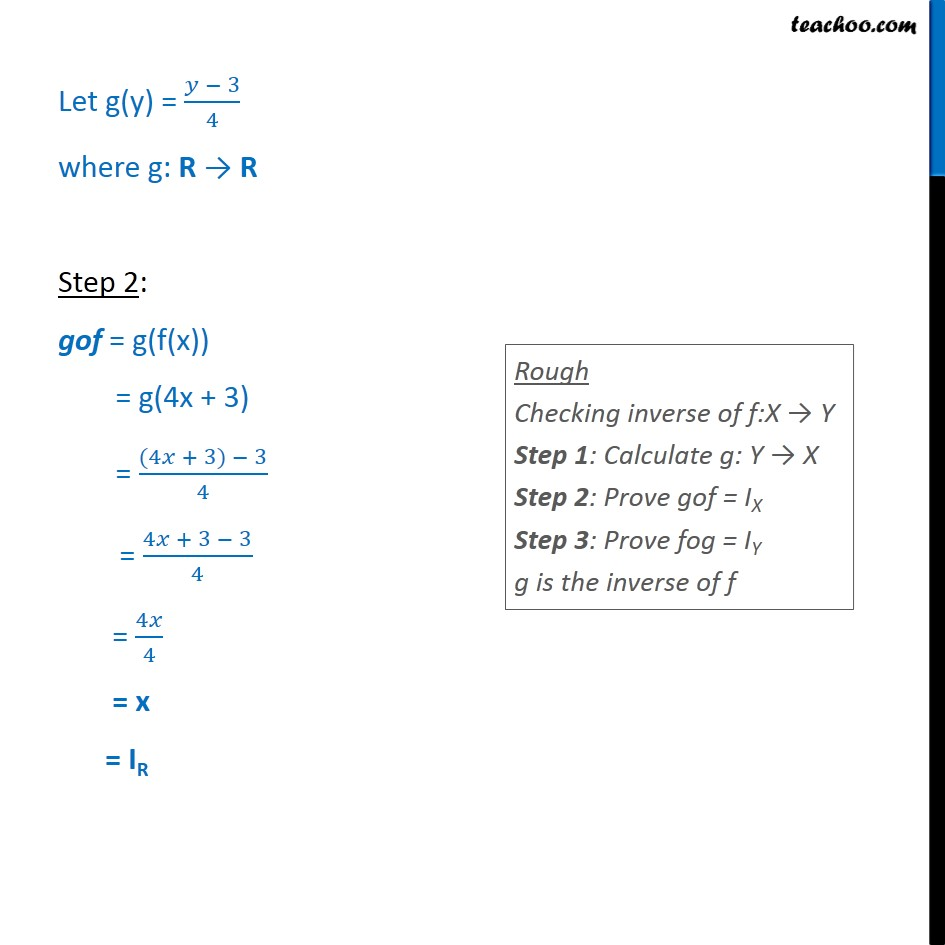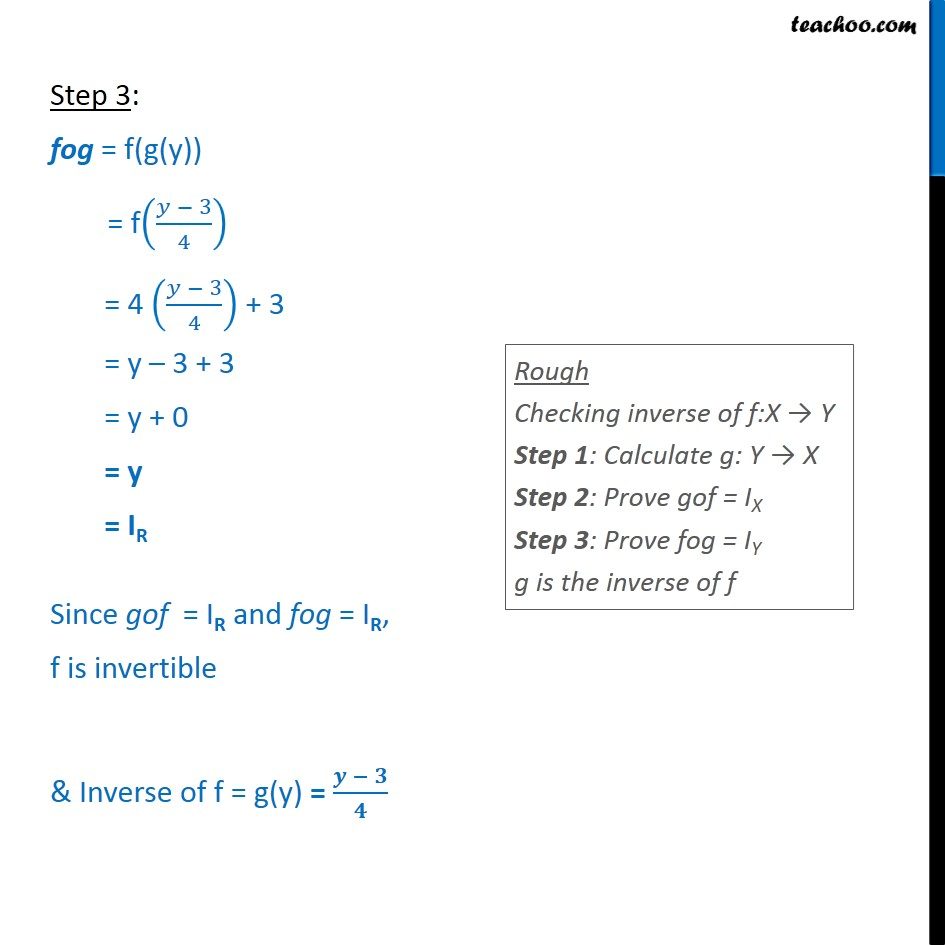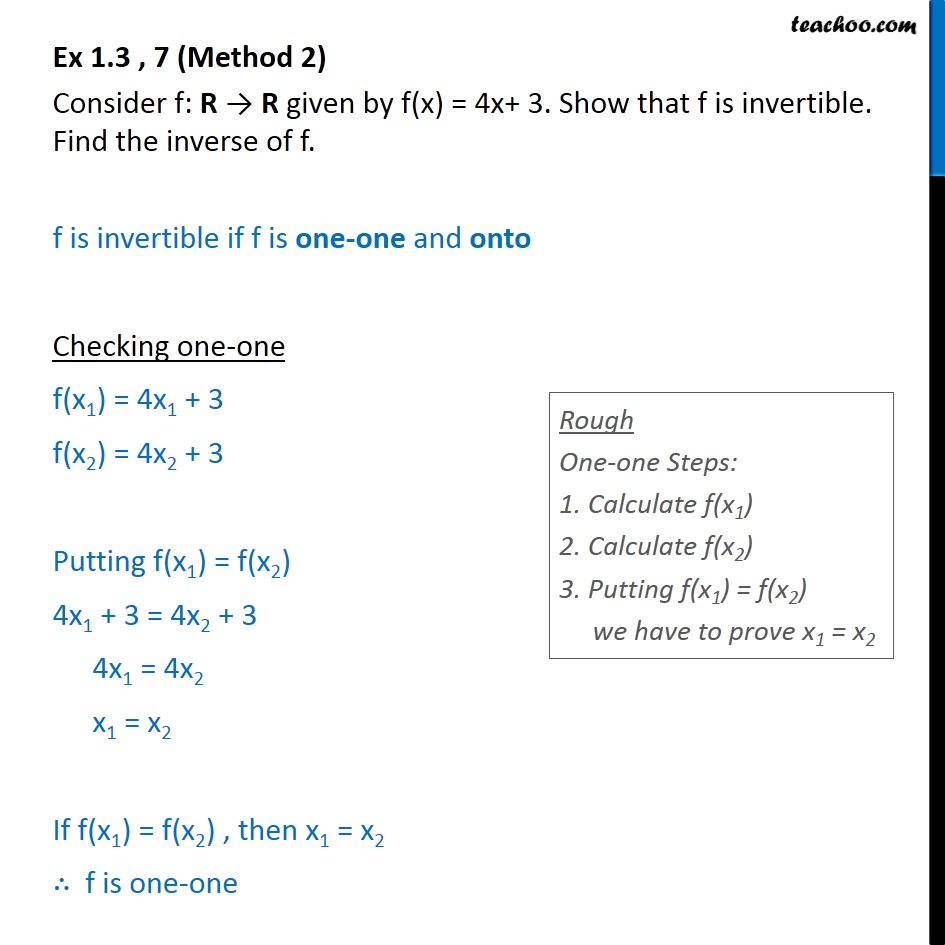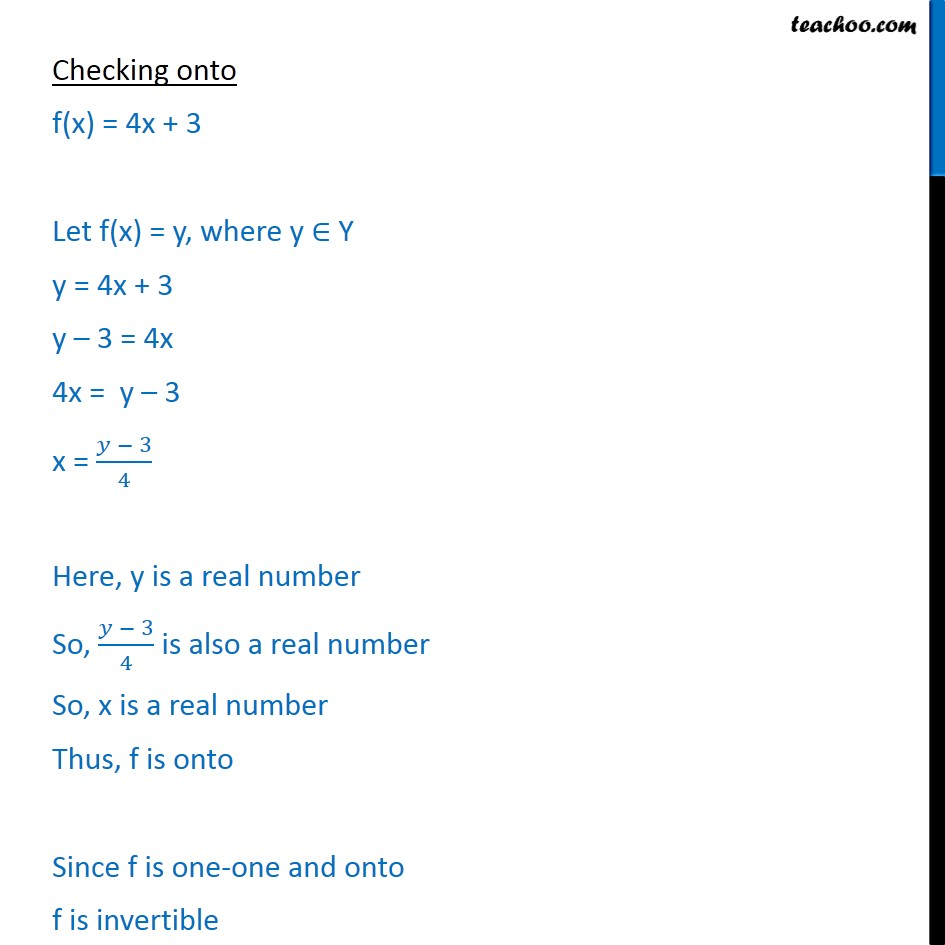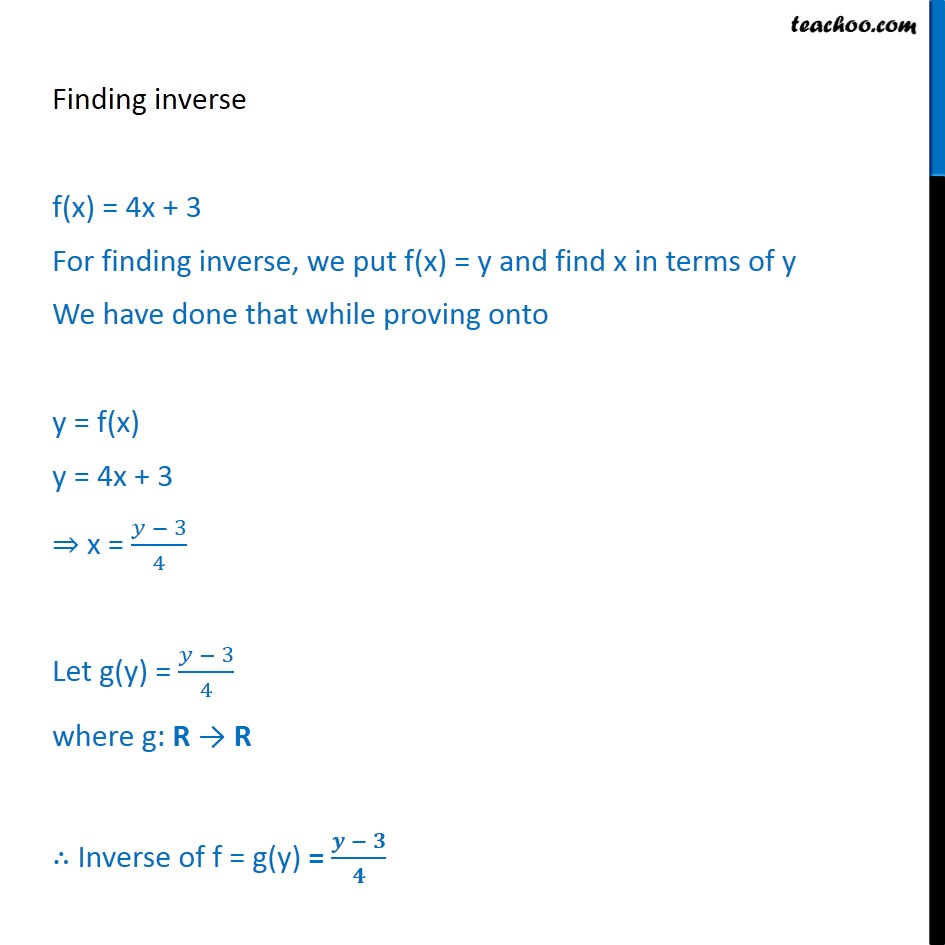Learn in your speed, with individual attention - Teachoo Maths 1-on-1 Class

### Transcript

Ex 1.3 , 7 (Method 1) Consider f: R R given by f(x) = 4x+ 3. Show that f is invertible. Find the inverse of f. Checking inverse Step 1 f(x) = 4x + 3 Let f(x) = y y = 4x + 3 y 3 = 4x 4x = y 3 x = 3 4 Let g(y) = 3 4 where g: R R Step 2: gof = g(f(x)) = g(4x + 3) = (4 + 3) 3 4 = 4 + 3 3 4 = 4 4 = x = IR Step 3: fog = f(g(y)) = f 3 4 = 4 3 4 + 3 = y 3 + 3 = y + 0 = y = IR Since gof = IR and fog = IR, f is invertible & Inverse of f = g(y) = Ex 1.3 , 7 (Method 2) Consider f: R R given by f(x) = 4x+ 3. Show that f is invertible. Find the inverse of f. f is invertible if f is one-one and onto Checking one-one f(x1) = 4x1 + 3 f(x2) = 4x2 + 3 Putting f(x1) = f(x2) 4x1 + 3 = 4x2 + 3 4x1 = 4x2 x1 = x2 If f(x1) = f(x2) , then x1 = x2 f is one-one Checking onto f(x) = 4x + 3 Let f(x) = y, where y Y y = 4x + 3 y 3 = 4x 4x = y 3 x = 3 4 Here, y is a real number So, 3 4 is also a real number So, x is a real number Thus, f is onto Since f is one-one and onto f is invertible Finding inverse f(x) = 4x + 3 For finding inverse, we put f(x) = y and find x in terms of y We have done that while proving onto y = f(x) y = 4x + 3 x = 3 4 Let g(y) = 3 4 where g: R R Inverse of f = g(y) =